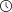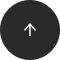- 7 minutes read

# The Most Helpful Python Data Cleaning Modules

Data cleaning is a critical part of data analysis. If you need to tidy a dataframe with Python, these will help you get the job done.

Python is the go-to programming language for data science. One reason it’s so popular is the rich selection of libraries. The functions and methods provided by these libraries expedite typical data science tasks.

Real-life data is usually messy and does not come in an appropriate format for data analysis. You are likely to spend lots of time cleaning and preprocessing the data before it is ready for analysis. Thus, it is crucially important to get familiar with Python’s data cleaning libraries. Our Introduction to Python for Data Science course provides a great overview of Python basics and introduces the fundamental Python libraries for data cleaning and dataframe tidying.

In this article, we will go over some of Python’s data cleaning libraries. Some of them are very commonly used, such as pandas and NumPy. In fact, Pandas might be the most popular Python library for data science. Some of the libraries we will cover are not as popular, but they come in handy for particular tasks.

### pandas

pandas is the most widely-used data analysis and manipulation library for Python. It provides numerous functions and methods for data cleaning. Its user-friendly syntax makes it easy to understand and implement solutions.

Dataframes are the core data structure of pandas; they store data in tabular form with labelled rows and columns. pandas is quite flexible in terms of manipulating dataframes, which is essential for an efficient data cleaning process.

You can easily add or drop columns or rows. Combining dataframes along rows or columns using the `concat` function is straightforward. In some cases, you will also need to collect data from multiple dataframes. The merge function is used for merging dataframes based on a shared column or columns.

Raw data may not always be in the optimal format. In such cases, you will need to create derived columns. You can apply basic aggregations on the existing columns to create new ones. pandas can perform such operations in a vectorized fashion, which makes it very fast. In addition to basic aggregations, pandas accepts user-defined functions or lambda expressions to preprocess existing columns.

Handling missing values is an essential part of data cleaning. It’s a two-step task: first you detect missing values, and then you replace them with appropriate values. `NA` and `NaN` are the standard missing value representations used by pandas. The `isna()` function returns `true` if a value in a cell is missing. You can combine the `isna()` and `sum()` functions to find the number of missing values in columns, rows, or the entire dataframe.

The second step is to fill the missing values. You should handle missing values carefully to keep data consistent. The `fillna()` function provides many different options to fill the missing values.

pandas is capable of handling not only numerical data but also textual data and dates. Its data-type-specific operations are grouped under accessors, which make it easier to learn them. The `str` accessor has several functions that manipulate strings. Similarly, the `dt` accessor provides several functions that manipulate dates and times.

Consider the following sample dataframe that contains name and age columns.If you want to show first and last names separately, the `split` function under the `str` accessor accomplishes this task in one line of code.

```df[['First_name', 'Last_name']] = df['Name'].str.split(' ', expand=True)
```

Here is how the dataframe looks now:Let’s also do an example with the `dt` accessor. In some cases, a certain part of a date needs to be extracted. For instance, you may need the month or day of the week information to be separate.We can easily extract the month and day of the week and assign them to new columns.

```df['month'] = df.col_a.dt.month
df['dayofweek'] = df.col_a.dt.dayofweek
```You can learn more about pandas on its official website. Its documentation pages are a good starting point, as they contain a lot of examples.

### NumPy

NumPy is a scientific computing library for Python and a fundamental library for the data science ecosystem. Some popular libraries are built on NumPy, including pandas and Matplotlib.

In recent years, it has become tremendously easy to both collect and store data. We are likely to work with substantial amounts of data. Thus, an efficient computing library is essential for data cleaning and manipulation.

NumPy offers us computationally efficient functions and methods. Its syntax is easy to grasp. The power of NumPy becomes more noticeable when working with multi-dimensional arrays.

### Matplotlib

Matplotlib is best known as a data visualization library, but it is also useful for data cleaning. You can create distribution plots, which help us better understand the data. In order to build an accurate and robust strategy to handle missing values, it is of great importance to have a comprehensive understanding of the underlying structure of the data.

The following figure is a histogram, which divides the value range of continuous variables into discrete bins and shows how many values are in each bin. It may provide useful information for data cleaning.### missingno

I mentioned the importance of handling missing values; the `missingno` library is a very handy tool for this task. It provides informative visualizations about the missing values in a dataframe.

For instance, you can create a missing value matrix that displays an overview of the missing value positions in the dataframe. Then you’ll be able to spot the areas with lots of missing values.

The following figure shows a missing value matrix. The white horizontal lines indicate the missing values. You can easily notice their distribution, which is an important insight for your strategy to handle the missing values.Here we can see that we've got a lot of missing data in the first column and even more in the third column.

The missingno library also provides a heatmap and a bar chart for displaying the missing values.

The library can be installed with pip using the following command:

```pip install missingno
```

### datacleaner

`datacleaner` is a third-party package that works with Pandas dataframes. What it does can also be achieved with Pandas, but datacleaner offers a succinct method that combines a few typical operations. In that sense, it saves both time and effort.

datacleaner can perform the following operations:

• Drop rows with missing values.
• Replace missing values with an appropriate value.
• Encode categorical variables.

### Modin

Modin can be considered as a pandas performance booster. It distributes data and computation to speed up the pandas code. According to Modin’s documentation, this can increase pandas’ speed by up to 4 times.

What I like best about Modin is its smooth integration with pandas. It does not add any unnecessary complexity to pandas’ syntax. You import Modin, replacing the regular pandas import, and then you are ready to go:

```import modin.pandas as pd
```

### PrettyPandas

PrettyPandas extends the pandas DataFrame class so you can customize how dataframes are displayed. As its name suggests, PrettyPandas makes dataframes look better.

PrettyPandas allows you to create tables that can be put directly into reports. You can easily add percentage and currency signs in the cells. Another useful feature is that the total and average values of columns can be displayed along with the table.

Consider the following pandas dataframe:After installing PrettyPandas with `pip`, we can import it and use it for customizing this dataframe. The following code block adds percentage signs to the first column and currency signs to the second and third columns. With `.total()` and `.average()`, we quickly add summary rows to our table.

```from prettypandas import PrettyPandas

(
df
.pipe(PrettyPandas)
.as_percent(subset = 'col_a')
.as_currency('USD', subset = 'col_b')
.as_currency('GBP', subset = 'col_c')
.total()
.average()
)
```

Here is how the dataframe looks now: